# Several Existence Theorems of Multiple Positive Solutions of Nonlinear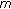-Point BVP for an Increasing Homeomorphism and Homomorphism on Time Scales

## Abstract

By using fixed point theorems in cones, the existence of multiple positive solutions is considered for nonlinear-point boundary value problem for the following second-order boundary value problem on time scales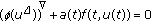,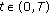,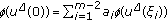,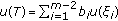, where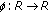is an increasing homeomorphism and homomorphism and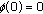. Some new results are obtained for the existence of twin or an arbitrary odd number of positive solutions of the above problem by applying Avery-Henderson and Leggett-Williams fixed point theorems, respectively. In particular, our criteria generalize and improve some known results by Ma and Castaneda (2001). We must point out for readers that there is only the-Laplacian case for increasing homeomorphism and homomorphism. As an application, one example to demonstrate our results is given.

## Author information

Authors

### Corresponding author

Correspondence to Shugui Kang.

## Rights and permissions

Reprints and Permissions

Han, W., Kang, S. Several Existence Theorems of Multiple Positive Solutions of Nonlinear-Point BVP for an Increasing Homeomorphism and Homomorphism on Time Scales. Bound Value Probl 2009, 584145 (2009). https://doi.org/10.1155/2009/584145

• Accepted:

• Published:

• DOI: https://doi.org/10.1155/2009/584145

### Keywords

• Differential Equation
• Partial Differential Equation
• Ordinary Differential Equation
• Functional Equation